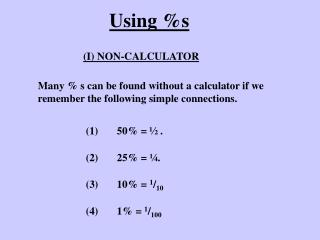DownloadDownload PresentationUsing %s

# Using %s

Télécharger la présentation## Using %s

- - - - - - - - - - - - - - - - - - - - - - - - - - - E N D - - - - - - - - - - - - - - - - - - - - - - - - - - -
##### Presentation Transcript

1. Using %s (I) NON-CALCULATOR Many % s can be found without a calculator if we remember the following simple connections. (1) 50% = ½ . (2) 25% = ¼. (3) 10% = 1/10 (4) 1% = 1/100

2. Ex1 To find 15% of 160kg ……… 10% of 160kg = 16kg 5% of 160kg = 8kg So 15% of 160kg = 24kg Ex2 To find 55% of £4200 ……… 50% of £4200 = £2100 5% of £4200 = £210 So 55% of £4200 = £2310

3. Ex3 To find 371/2% of 160kg ……… 25% of 160kg = 40kg 121/2% of 160kg = 20kg So 371/2% of 160kg = 60kg Ex4 To find 31/2% of £4200 ……… 1% of £4200 = £42 3% of £4200 = £126 1/2% of £4200 = £21 So 31/2% of £4200 = £147

4. Exercise 10% of £64 = £6.40 (1) 171/2% of £64 …. 5% of £64 = £3.20 21/2% of £64 = £1.60 So 171/2% of £64 = £11.20 (2)621/2% of £56.80 …. 50% of £56.80 = £28.40 25% of £56.80 = £14.20 121/2% of £56.80 = £7.10 So621/2% of £56.80 = £35.50

5. USING A CALCULATOR Percentages & Decimals Simple Rule - Must be Learned 23% = 0.23, 77% = 0.77, 6% = 0.06, NB: 60% = 0.60 or 0.6 Conversely 0.78 = 78%, 0.41 = 41%, 0.07 = 7%, 0.025 = 2.5%

6. Example 34% of £45.50 = 0.34 X £45.50 = £15.47 Example A bank pays interest of 3.7% per annum (yearly). Find the interest paid on £1290. £47.73 Interest = 3.7% of £1290 = 0.037 X £1290 =

7. % Reductions Example In a sale a TV set that normally costs £380 is reduced by 35%. Find the sale price. Long Method Saving = 35% of £380 = 0.35 X £380 = £133 Sale price = £380 - £133 = £247 Short Method (by multiplier) NB: removing 35% leaves 65% or 0.65 Sale price = 0.65 X £380 = £247

8. Timed Questions Copy & complete the following Ex1 Hi-fi - usual price £650 - Saving = 30% % paid = 70% Cost = 70% of £650 = 0.7 X £650 = £455 Ex2 Sofa - usual price £1200 - Saving 42% % paid = 58% Cost = 58% of £1200 = 0.58 X £1200 = £696

9. Exercise Now try the following setting out the answers the same way. (1) DVD Player: usual price = £280 Saving = 22% % paid = 78% Cost = 78% of £280 = 0.78 X £280 = £218.40 (2) Bed: usual price = £475 Saving = 34% % paid = 66% Cost = 66% of £475 = 0.66 X £475 = £313.50 (3) Wig: usual price £160 Saving 19% % paid = 81% Cost = 81% of £160 = 0.81 X £160 = £129.60

10. Exercise Now try the following setting out the answers the same way. (4) CD Player: usual price = £190 Saving = 32% % paid = 68% Cost = 68% of £190 = 0.68 X £190 = £129.20 (5) Fridge: usual price = £470 Saving = 18% % paid = 82% Cost = 82% of £470 = 0.82 X £470 = £385.40 (6) Cinema ticket: usual price £4.80 Saving 20% % paid = 80% Cost = 80% of £4.80 = 0.80 X £4.80 = £3.84

11. (7) Jeans: usual price = £37.50 Saving = 28% % paid = 72% Cost = 72% of £37.50 = 0.72 X £37.50 = £27 (8) Calculator: usual price = £8.95 Saving = 31% % paid = 69% Cost = 69% of £8.95 = 0.69 X £8.95 = £6.18 (9) Fish Supper: usual price £4.35 Saving 44% % paid = 56% Cost = 56% of £4.35 = 0.56 X £4.35 = £2.44

12. Important Point Increasing a quantity by 12% gives us 112% of what we had already, and 112% = 1.12. Increasing a quantity by 8% gives us 108% of what we had already, and 108% = 1.08. This can now be used as follows …..

13. % Increasess Example A waitress earns £4.20 an hour but gets a 5% pay rise. Find her new pay rate. Long Method Rise = 5% of £4.20 = 0.05 X £4.20 = £0.21 New rate = £4.20 + £0.21 = £4.41 Short Method (by multiplier) NB: adding 5% gives 105% or 1.05 New rate = 1.05 X £4.20 = £4.41

14. Timed Questions Copy & complete the following Ex1 Joiner - usual rate £9.50 per hour - Increase = 12% New % = 112% New rate = 112% of £9.50 = 1.12 X £9.50 = £10.64 Ex2 Town population = 12000 - Increase 43% New % = 143% New population = 143% of 12000 = 1.43 X 12000 = 17160